``````x=0:2*pi;
y=sin(x);
xx=0:0.5:2*pi;

%interp1对sin函数进行分段线性插值，调用interp1的时候，默认的是分段线性插值
y1=interp1(x,y,xx);
figure
plot(x,y,'o',xx,y1,'r')
title('分段线性插值')

%临近插值
y2=interp1(x,y,xx,'nearest');
figure
plot(x,y,'o',xx,y2,'r');
title('临近插值')

%球面线性插值
y3=interp1(x,y,xx,'spline');
figure
plot(x,y,'o',xx,y3,'r')
title('球面插值')

%三次多项式插值法
y4=interp1(x,y,xx,'cubic');
figure
plot(x,y,'o',xx,y4,'r');
title('三次多项式插值')``````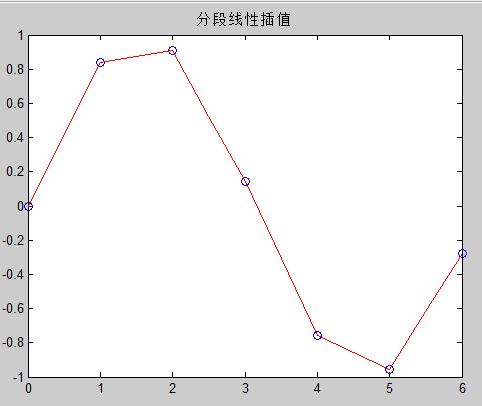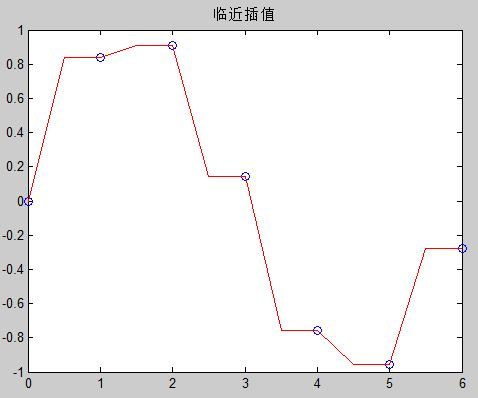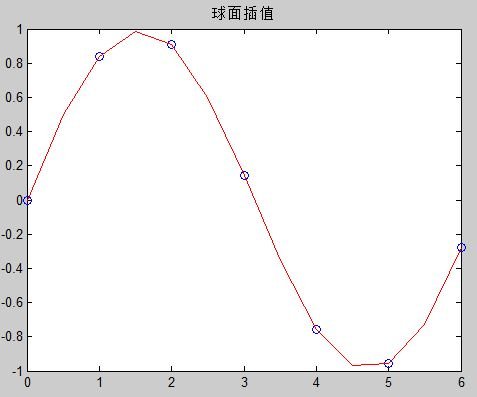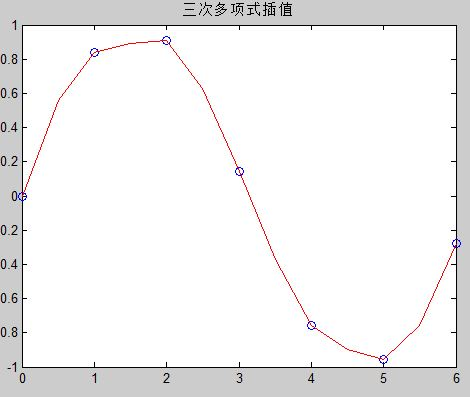(1)    Nearest方法速度最快，占用内存最小，但一般来说误差最大，插值结果最不光滑。

(2)    Spline三次样条插值是所有插值方法中运行耗时最长的，插值函数及其一二阶导函数都连续，是最光滑的插值方法。占用内存比cubic方法小，但是已知数据分布不均匀的时候可能出现异常结果。

(3)    Cubic三次多项式插值法中，插值函数及其一阶导数都是连续的，所以插值结果比较光滑，速度比Spline快，但是占用内存最多。

 语法形式 说明 y=interp1(x,Y,xi) 由已知点集(x,Y)插值计算xi上的函数值 y=interp1(x,Y,xi) 相当于x=1:length(Y)的interp(x,Y,xi) y=interp1(x,Y,xi,method) 用指定插值方法计算插值点xi上的函数值 y=interp1(x,Y,xi,method,’extrap’) 对xi中超出已知点集的插值点用指定插值方法计算函数值 y=interp1(x,Y,xi,method,’extrap’,extrapval) 用指定方法插值xi上的函数值，超出已知点集处函数值取extrapval y=interp1(x,Y,xi,method,’pp’) 用指定方法插值，但返回结果为分段多项式

 Method 方法描述 ‘nearest’ 最邻近插值:插值点处函数值与插值点最邻近的已知点函数值相等 ‘liner’ 分段线性插值：插值点处函数值由连接其最邻近的两侧点的线性函数预测。Matlab中interp1的默认方法。 ‘spline’ 样条插值：默认为三次样条插值。可用spline函数替代 ‘pchip’ 三次Hermite多项式插值，可用pchip函数替代 ‘cubic’ 同’pchip’，三次Hermite多项式插值

``pp = interp1(x,v,method,'pp')``

``````%test interpolate
clear;clc;close all
N=1200;
x = 1:0.5:6;
y = sin(x);
pp = interp1(x,y,'pchip','pp');

xq= 1:0.1:6;
result = ppval(pp,xq);
plot(x,y,'o',xq,result,'-.')``````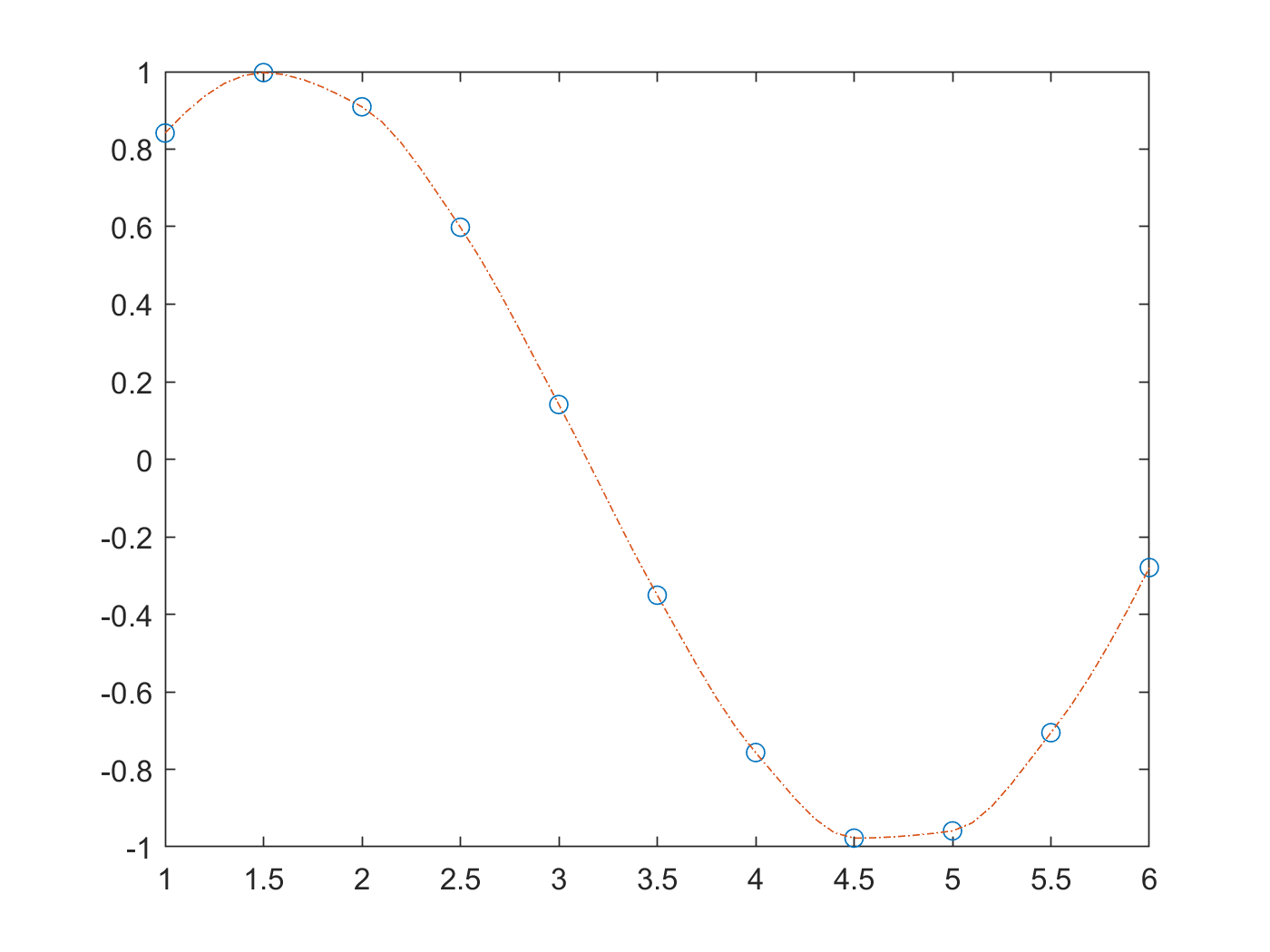ModelScope旨在打造下一代开源的模型即服务共享平台，为泛AI开发者提供灵活、易用、低成本的一站式模型服务产品，让模型应用更简单！